• 仿真kalman滤波在2D目标运动中的应用，包含扩展kalman滤波与无迹kalman滤波
• kalman滤波的仿真-kalman滤波的仿真.rar kalman方面的代码，以及kalman滤波的仿真，与工具箱matlab
• 实现KALMAN滤波算法通过跟踪估计物体运动轨迹-kalman滤波.rar 非常好得KALMAN滤波算法，通过跟踪，估计物体运动轨迹，和大家一起分享。 所含文件： Figure2.jpg KALMAN滤波算法，通过跟踪，...matlab
• 简要介绍了kalman 滤波的原理和算法 ，包含matlab 程序源代码
• 一维kalman滤波的程序，解决线性kalman滤波问题
• kalman滤波一阶模型 带有详细的注释 通过测试
• Kalman滤波： 其中 A~k~为状态转移矩阵， R为状态估计噪声， Q为观测噪声， C~k~为观测矩阵,， P~k~为状态的协方差，需要对 P~0~初始化。 Extended Kalman滤波： 预测： 更新卡尔曼增益： 计算后验概率...
Kalman滤波：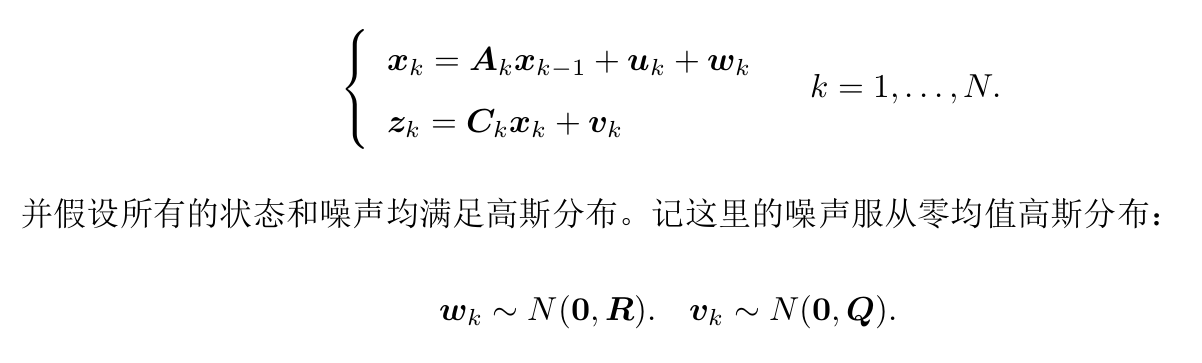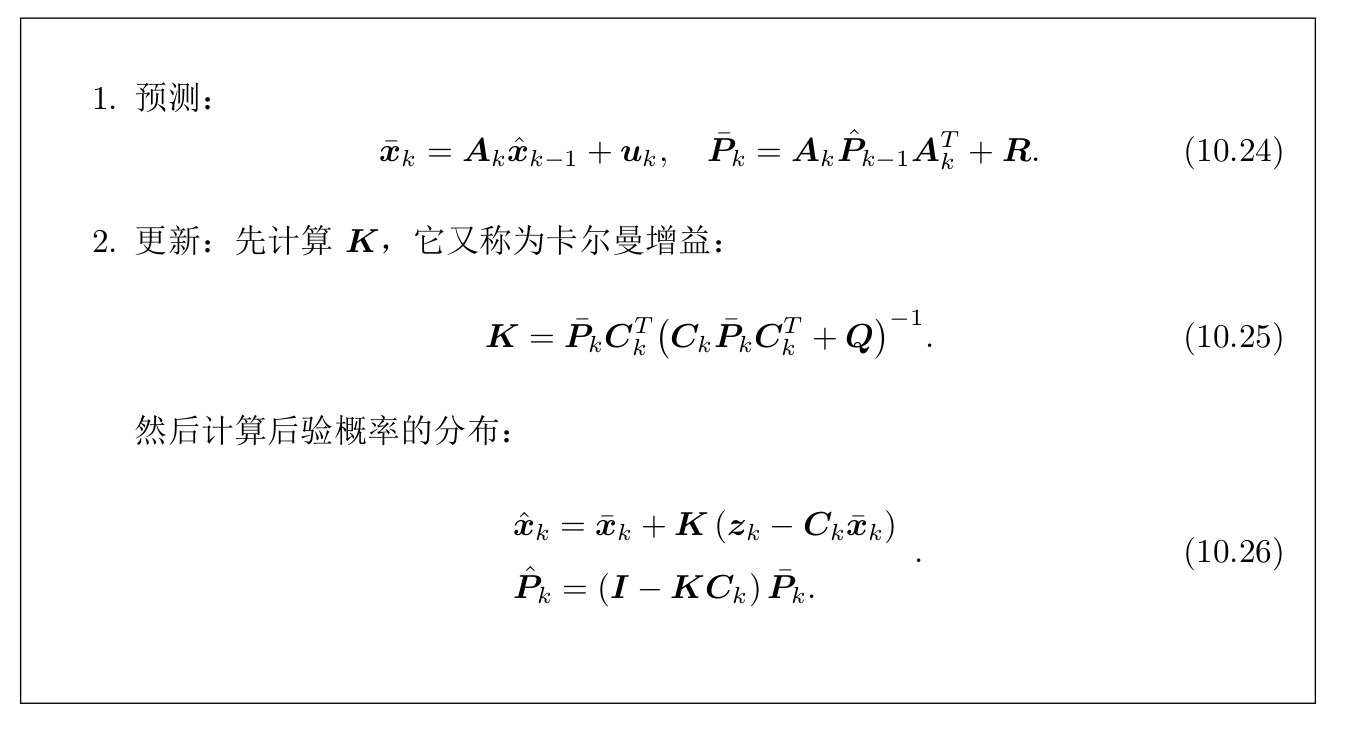其中 Ak为状态转移矩阵， R为状态估计噪声， Q为观测噪声， Ck为观测矩阵,， Pk为状态的协方差，需要对 P0初始化。

Extended Kalman滤波：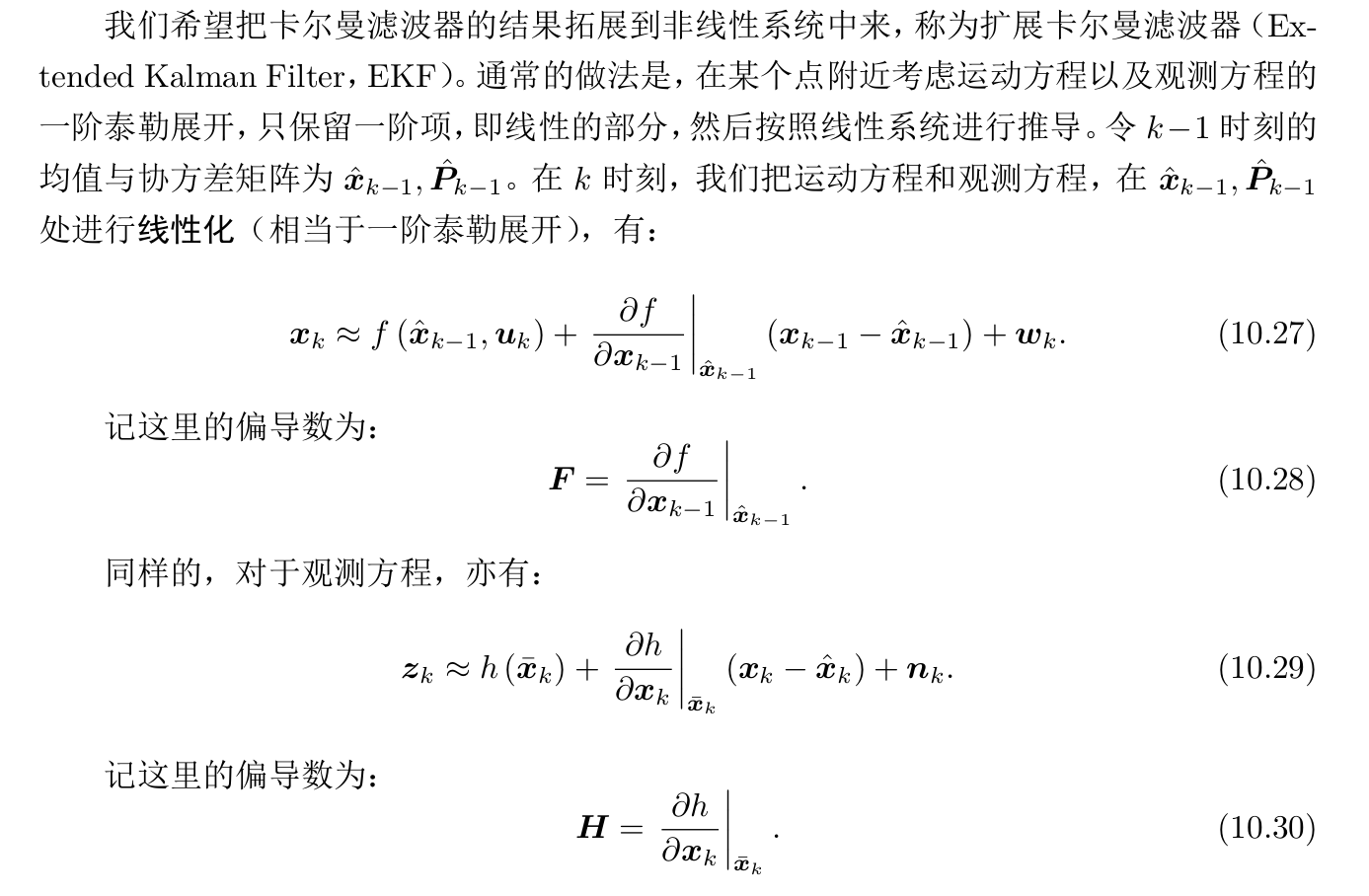预测：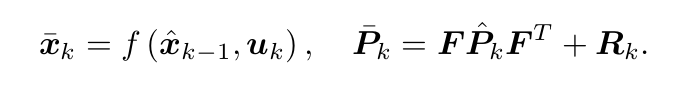更新卡尔曼增益：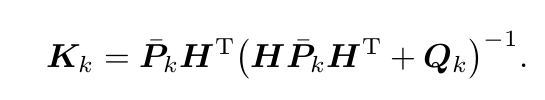计算后验概率分布：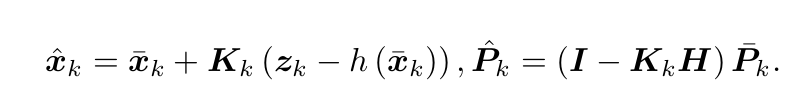两个高斯分布乘积的理论推导[链接](https://www.cnblogs.com/rainbow70626/p/14070485.html)


展开全文自动驾驶
• 文章目录Kalman 滤波1. 简介2. 极大似然估计3. 状态空间参考 Kalman 滤波 1. 简介 Kalman 滤波常用于目标的追踪、预测等任务。滤波器被设计为最小化均方误差。此外，还可以用极大似然的方法得到滤波器。滤波器就是...
文章目录Kalman 滤波1. 简介2. 极大似然估计3. 状态空间参考
Kalman 滤波
1. 简介
Kalman 滤波常用于目标的追踪、预测等任务。滤波器被设计为最小化均方误差。此外，还可以用极大似然的方法得到滤波器。滤波器就是用来从信号中提取出有用的信息，使用一个损失函数评价滤波器的性能。
2. 极大似然估计
使用极大似然的方法得到一个滤波器，最终的目标就是得到一个$\hat{x}$ 使得$y$的条件概率最大，即
$max [P(y|\hat{x})]$
假设信号中的噪声是服从高斯分布的$\mathcal{N}(0,\sigma_k)$。假设信号为
$y_k=a_kx_k+n_k$
其中$n_k$是噪声，$x_k$是承载数据的信号，$a_k$是系数，那么根据噪声服从高斯分布以及条件概率公式可以得到：
$P(y_k|\hat{x}_k)=K_k \cdot e^{-\left(\frac{(y_k-a_k\hat{x_k})^2}{2\sigma_k^2}\right)}$
对上式求对数得到：
$\log P(y|\hat{x})=-\frac{1}{2}\sum_{k}\left(\frac{(y_k-a_k\hat{x}_k)^2}{\sigma_k^2}\right)+C$
其中$C$是常数项。
3. 状态空间
假设目标是要获得如下过程中变量的值：
$x_{k+1}=\Phi x_k+\omega_k$
其中 $x_k$是$t$时刻的状态向量，$\Phi$是从时刻 $t$到时刻 $t+1$的状态转移矩阵，并且是被认为时间平稳的，$\omega_k$是高斯白噪声。
变量的观察量定义为：
$z_k=H x_k+v_k$
其中 $z_k$是时刻$t$的$x$的观测量，$H$是将状态向量转换为观测向量的矩阵，并且被认为是时间平稳的，$v_k$是度量误差，是高斯白噪声。
两个噪声的协方差被认为是时间平稳的，分别表示为：
$Q=E[\omega_k \omega_k^T]\\ R=E[v_kv_k^T]$
将$x_k$的估计表示为 $\hat{x}_k$，使用均方误差衡量滤波器的性能，并表示为
$f(e_k)=(x_k-\hat{x}_k)^2$

设$P_k=E[e_ke_k^T]=E\left[(x_k-\hat{x}_k)(x_k-\hat{x}_k)^T\right]$，并将之前一次的估计记为$\hat{x}'_k$，那么根据历史估计以及误差情况得到下面这个等式：
$\hat{x}_k=\hat{x}'_k+K_k(z_k-H\hat{x}'_k)$
其中 $K_k$是Kalman 增益值，后面给出推导公式，后面一项$z_k-H\hat{x}'_k$为测量残留(measurement residual)。
将$z_k$的定义替换到上式中得到：
$\hat{x}_k=\hat{x}'_k+K_k(Hx_k+v_k-H\hat{x}'_k)$
再将$\hat{x}_k$带入到上式$P_k$的等式中得到：
\begin{aligned} &P_k=E\left[\left[(I-K_kH)(x_k-\hat{x}_k')-K_kv_k\right]\left[(I-K_kH)(x_k-\hat{x}_k')\right]\right]\\ &=(I-K_kH)E\left[(x_k-\hat{x}_k')(x_k-\hat{x}_k')^T\right](I-K_kH)+K_kE[v_kv_k^T]K_k^T \end{aligned}
将之前的预测$P_k'$以及$R$替换上面等式中的均方误差项以及噪声的协方差项得到：
$P_k=(I-K_kH)P_k'(I-K_kH)^T+K_kRK_k^T$
对于矩阵 $P_k$，其对角线上包含均方误差项，因此最小化均方误差的目标可以转换为最小化矩阵 $P_k$的迹(trace)。
首先将上式展开得到

$P_k=P_k'-K_kHP_k'-P_k'H^TK_k^T+K_k(HP_k'H^T+R)K_k^T$
设$T[]$表示矩阵的迹，则上式两边表示为
$T[P_k]=T[P'_k]-2T[K_kHP_k']+T[K_k(HP_k'H^T+R)K_k^T]$
对$K_k$求导得到：
$\frac{dT[P_k]}{dK_k}=-2(HP_k')^T+2K_k(HP_k'H^T+R)$
并令其等于0，得到：
$(HP'_k)^T=K_k(HP_k'H^T+R)$
并最终得到Kalman增益等式：
$K_k=P_k'H^T(HP_k'H^T+R)^{-1}$
将该等式带入到上面$P_k$的等式中，得到：
\begin{aligned} &P_k=P_k'-P_k'H^T(HP_k'H^T+R)^{-1}HP_k'\\ &=P_k'-K_kHP_k'\\ &=(I-K_kH)P_k' \end{aligned}
前面已经定义了关于变量$x_k$从时刻$k$到时刻 $k+1$的转移：
$x_{k+1}'=\Phi\hat{x}_k$
对于均方误差中的 $e_k$，更新方法为：
\begin{aligned} &e_{k+1}'=x_{k+1}-\hat{x}'_{k+1}\\ &=(\Phi x_k+\omega_k)-\Phi \hat{x}_k\\ &=\Phi e_k+\omega_k \end{aligned}
和前面$P_k$的等式类似，$k+1$时刻：
\begin{aligned} &P_{k+1}'=E[e^{'}_{k+1} e_{k+1}^{'T}]\\ &= E\left[\Phi e_k(\Phi e_k)^T\right]+E[\omega_k \omega_k^T]\\ &=\Phi P_k\Phi^T+Q \end{aligned}
算法的整体流程如下图所示：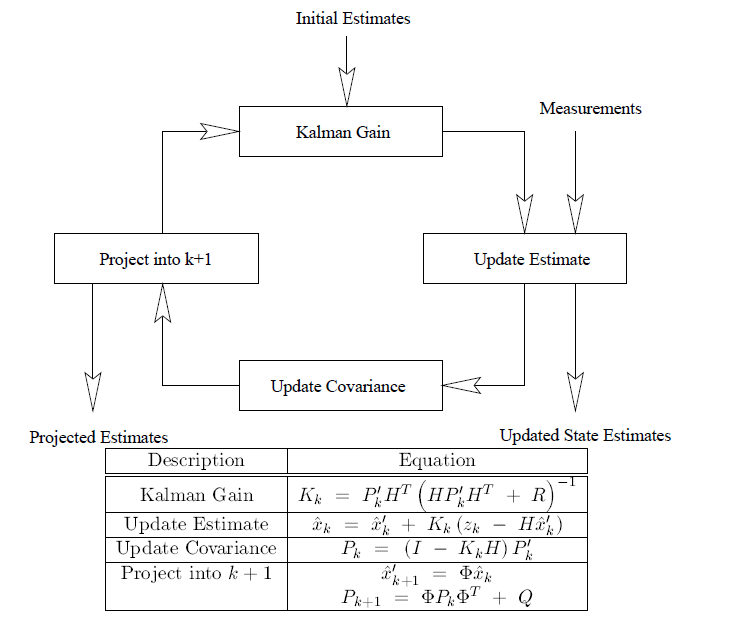参考
 The Kalman filter


展开全文• Kalman滤波算法Kalman滤波算法Kalman滤波算法Kalman滤波算法
• 内含两篇论文，一篇是Kalman老先生1960年关于Kalman滤波开山之作，一篇2012年的论文，非常生动解释了Kalman滤波的思想。
• 卡尔曼滤波教程：kalman滤波全揭秘卡尔曼滤波教程：kalman滤波全揭秘卡尔曼滤波教程：kalman滤波全揭秘
• 离散Kalman滤波理论及其应用，刘玉婵，张书毕，本文简单介绍了离散Kalman滤波的基本思想及状态方程和观测方程，叙述了解算 Kalman滤波的5个步骤及其公式，为了加深对离散Kalman滤波的�
• 数据滤波是去除噪声还原真实数据的一种数据处理技术，Kalman滤波在测量方差已知的情况下能够从一系列存在测量噪声的数据中，估计动态系统的状态。由于它便于计算机编程实现，并能够对现场采集的数据进行实时的更新和...
• 一维kalman滤波代码，有详细的代码注释。通过简单的例子入门kalman滤波计算方法
• 基于kalman滤波的meanshift算法 代码可供参考 效果一般 基于kalman滤波的meanshift算法 代码可供参考 效果一般...